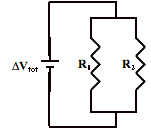# Electricity: Electric Ciruits

## Electric Circuits: Audio Guided Solution

#### Problem 30:The parallel circuit at the right depicts two resistors connected to a voltage source. The voltage source (ΔVtot) is a 12-V source and the resistor values are 6.4 Ω (R1) and 3.9 Ω (R2).
a. Determine the equivalent resistance of the circuit.
b. Determine the current in each branch resistor.
c. Determine the total current in the circuit.

## Audio Guided Solution

Click to show or hide the answer!

## Habits of an Effective Problem Solver

• Read the problem carefully and develop a mental picture of the physical situation. If necessary, sketch a simple diagram of the physical situation to help you visualize it.
• Identify the known and unknown quantities and record them in an organized manner. Equate given values to the symbols used to represent the corresponding quantity - e.g., ΔV = 9.0 V; R = 0.025 Ω; I = ???.
• Use physics formulas and conceptual reasoning to plot a strategy for solving for the unknown quantity.
• Identify the appropriate formula(s) to use.
• Perform substitutions and algebraic manipulations in order to solve for the unknown quantity.